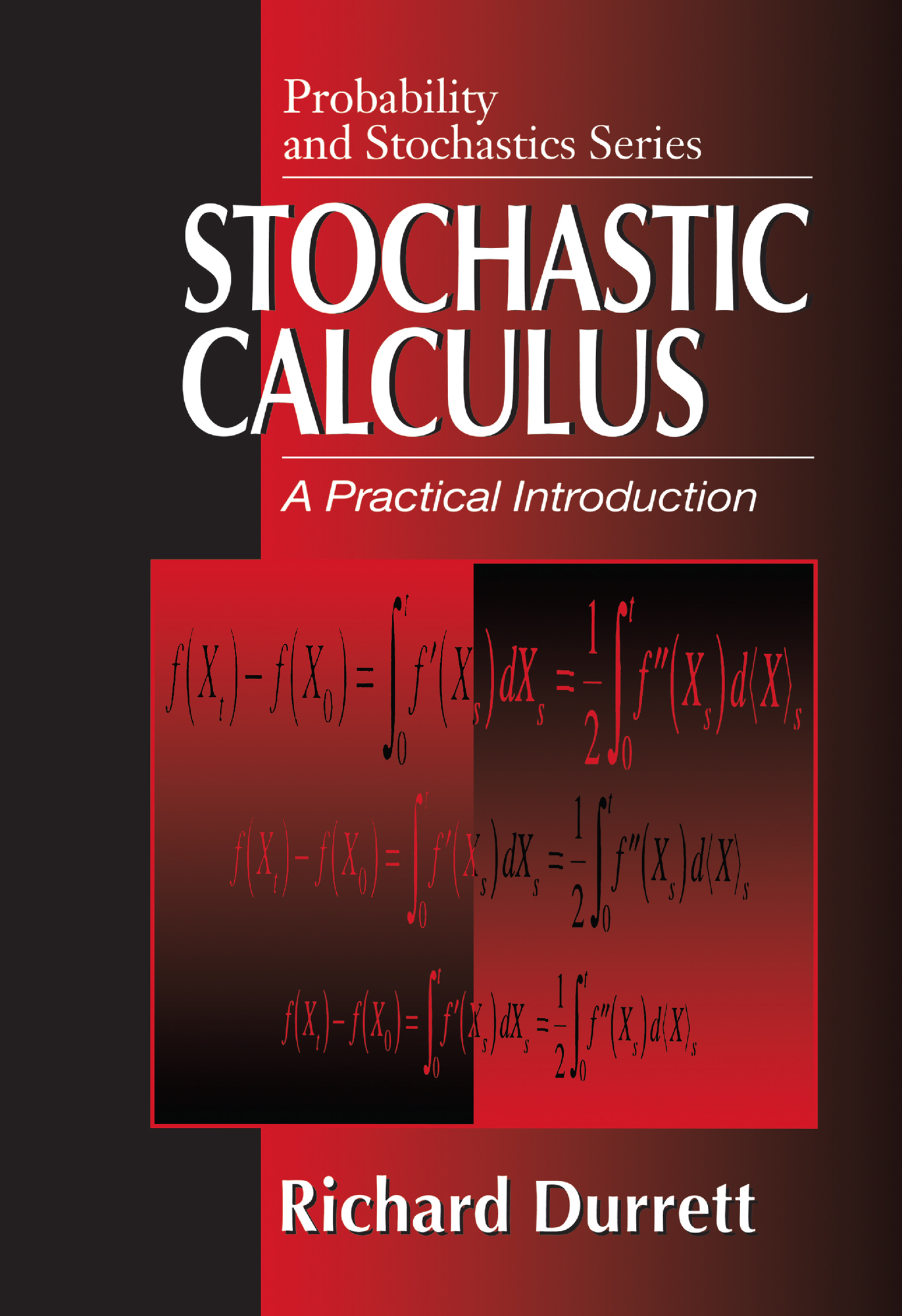Stochastic Calculus

A Practical Introduction, 1st Edition

CRC Press

341 pages

Purchasing Options:\$ = USD
Hardback: 9780849380716
pub: 1996-06-21
SAVE ~\$34.00
\$170.00
\$136.00
x
eBook (VitalSource) : 9780203738283
pub: 2018-03-29
from \$28.98

FREE Standard Shipping!

Description

This compact yet thorough text zeros in on the parts of the theory that are particularly relevant to applications . It begins with a description of Brownian motion and the associated stochastic calculus, including their relationship to partial differential equations. It solves stochastic differential equations by a variety of methods and studies in detail the one-dimensional case. The book concludes with a treatment of semigroups and generators, applying the theory of Harris chains to diffusions, and presenting a quick course in weak convergence of Markov chains to diffusions.

The presentation is unparalleled in its clarity and simplicity. Whether your students are interested in probability, analysis, differential geometry or applications in operations research, physics, finance, or the many other areas to which the subject applies, you'll find that this text brings together the material you need to effectively and efficiently impart the practical background they need.

CHAPTER 1. BROWNIAN MOTION

Definition and Construction

Markov Property, Blumenthal's 0-1 Law

Stopping Times, Strong Markov Property

First Formulas

CHAPTER 2. STOCHASTIC INTEGRATION

Integrands: Predictable Processes

Integrators: Continuous Local Martingales

Variance and Covariance Processes

Integration w.r.t. Bounded Martingales

The Kunita-Watanabe Inequality

Integration w.r.t. Local Martingales

Change of Variables, Itô's Formula

Integration w.r.t. Semimartingales

Associative Law

Functions of Several Semimartingales

Chapter Summary

Meyer-Tanaka Formula, Local Time

Girsanov's Formula

CHAPTER 3. BROWNIAN MOTION, II

Recurrence and Transience

Occupation Times

Exit Times

Change of Time, Lévy's Theorem

Burkholder Davis Gundy Inequalities

Martingales Adapted to Brownian Filtrations

CHAPTER 4. PARTIAL DIFFERENTIAL EQUATIONS

A. Parabolic Equations

The Heat Equation

The Inhomogeneous Equation

The Feynman-Kac Formula

B. Elliptic Equations

The Dirichlet Problem

Poisson's Equation

The Schrödinger Equation

C. Applications to Brownian Motion

Exit Distributions for the Ball

Occupation Times for the Ball

Laplace Transforms, Arcsine Law

CHAPTER 5. STOCHASTIC DIFFERENTIAL EQUATIONS

Examples

Itô's Approach

Extension

Weak Solutions

Change of Measure

Change of Time

CHAPTER 6. ONE DIMENSIONAL DIFFUSIONS

Construction

Feller's Test

Recurrence and Transience

Green's Functions

Boundary Behavior

Applications to Higher Dimensions

CHAPTER 7. DIFFUSIONS AS MARKOV PROCESSES

Semigroups and Generators

Examples

Transition Probabilities

Harris Chains

Convergence Theorems

CHAPTER 8. WEAK CONVERGENCE

In Metric Spaces

Prokhorov's Theorems

The Space C

Skorohod's Existence Theorem for SDE

Donsker's Theorem

The Space D

Convergence to Diffusions

Examples

Solutions to Exercises

References

Index